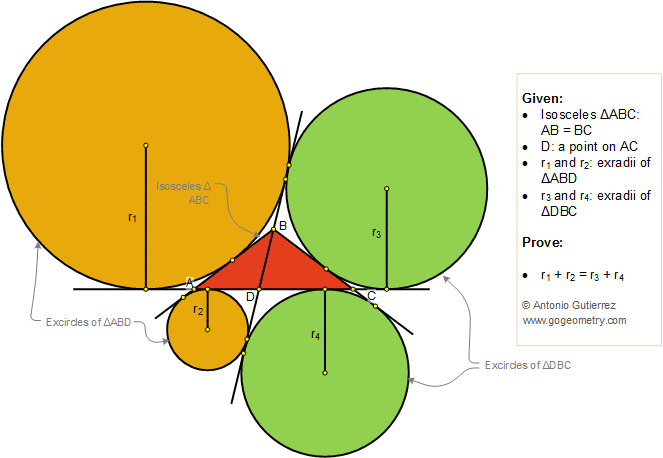# Geometry Problem 1377: Isosceles Triangle, Interior Cevian, Equal Sum of Exradii, Excircle.

### Proposition

In the figure below, ABC is an isosceles triangle (AB = BC) D is a point on AC, r1 and r2 are the exradii of the triangle ABD corresponding to AB and AD, respectively; r3 and r4 are the exradii of the triangle DBC corresponding to BC and CD, respectively. Prove that r1 + r2 = r3 + r4.

BD is called an interior cevian of triangle ABC.